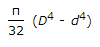# Civil Engineering - Theory of Structures

### Exercise :: Theory of Structures - Section 3

36.

In the cable shown in the given figure, the minimum tension occurs at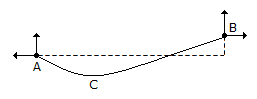A. A B. B C. C D. between A and C E. between B and C

Explanation:

No answer description available for this question. Let us discuss.

37.

A shaft rotating N.R.M. under a torque T, transmits a power

 A.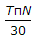Newton metres/sec B.Newton metres/min C.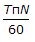Newton metres/min D.Newton metres/sec

Explanation:

No answer description available for this question. Let us discuss.

38.

In the truss shown in given figure, the force in member BD is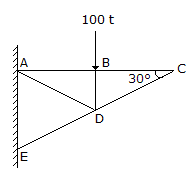A. 100 t compressive B. 100 t tensile C. zero D. indeterminate

Explanation:

No answer description available for this question. Let us discuss.

39.

The normal and tangential components of stress on an inclined plane through θ° to the direction of the force, will be equal if θ is

 A. 45° B. 30° C. 60° D. 90°

Explanation:

No answer description available for this question. Let us discuss.

40.

If D and d are external and internal diameters of a circular shaft respectively, its polar moment of inertia, is

 A.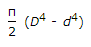B.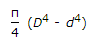C.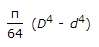D.